# KEPLER: A Unified Model for Knowledge Embedding and Pre-trained Language Representation

## Basic Idea

• 实体嵌入和语言分离, 不方便表示空间的对齐. KGE模型很少将KG结构作为输入, 并很少结合文本信息, 因此无法帮助PLM.
• 需要实体链接器, 在传播时容易出现错误.
• 与普通PLM相比, 查找实体的表示会带来额外的开销.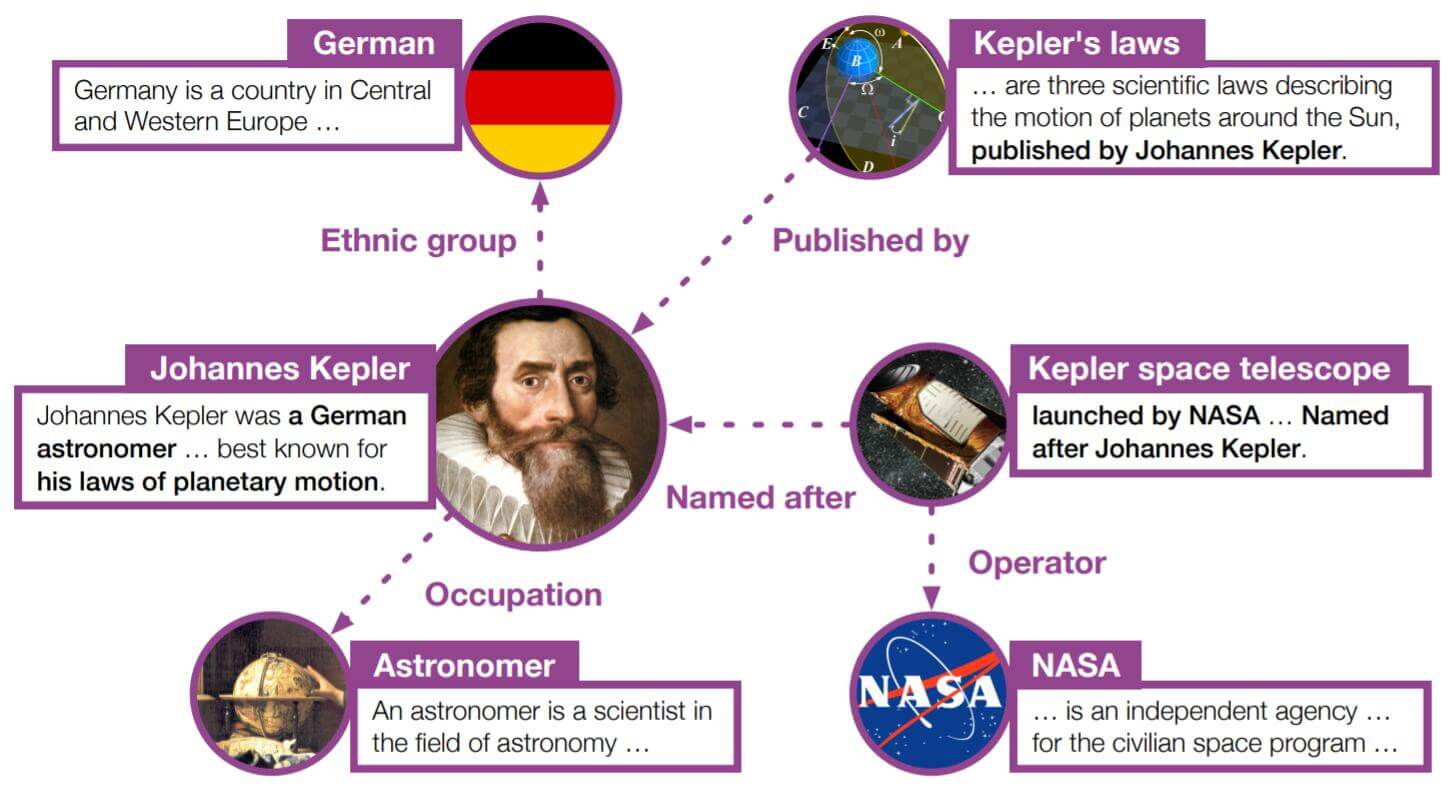## KEPLER

KEPLER(Knowledge Embedding and Pre - trained LanguagE Representation)是一个KGE和PLM表示统一的模型. 所以它包含了PLM和KE的联合优化目标.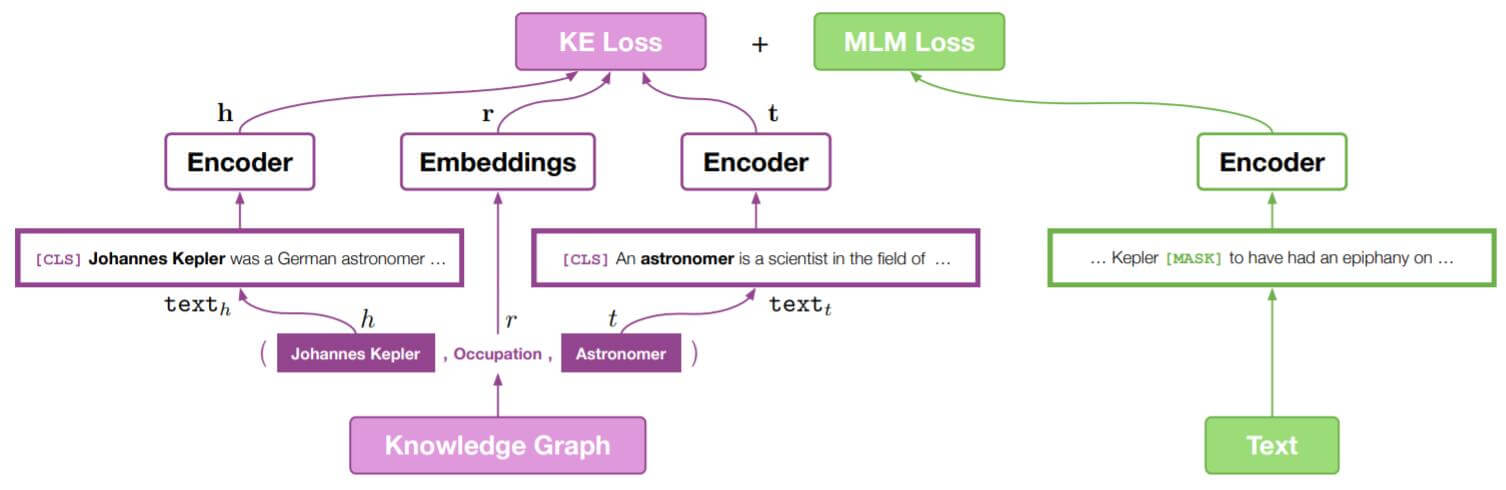### Encoder

$$\mathbf{H}_i=\mathrm{E}_i(\mathbf{H}_{i-1})$$

### Knowledge Embedding

• 只用实体描述.
• 使用实体描述关系描述.

#### Using Entity Descriptions

\begin{aligned} \mathbf{h} &=\mathrm{E}_{[\mathrm{CLS}]}\left(\operatorname{text}_{h}\right) \\ \mathbf{t} &=\mathrm{E}_{[\mathrm{CLS}]}\left(\operatorname{text}_{t}\right) \\ \mathbf{r} &=\mathbf{T}_{r} \end{aligned}

#### Using Entity and Relation Descriptions

$$\mathbf{h}_{r}=\mathrm{E}_{[\mathrm{CLS}]}\left(\operatorname{text}_{h, r}\right)$$

1. 头实体获得关系描述加成(也有可能是污染), 尾实体没有被考虑到.
2. BERT捕捉上下文的能力被局限了, 仅能体现在描述的上下文当中, 并不能根据语境调整实体的表示.

#### Konwledge Embedding Loss and Score Function

KE部分的损失如下:

$$\mathcal{L}_{\mathrm{KE}} =-\log \sigma\left(\gamma-d_{r}(\mathbf{h}, \mathbf{t})\right) -\sum_{i=1}^{n} \frac{1}{n} \log \sigma\left(d_{r}\left(\mathbf{h}_{\mathbf{i}}^{\prime}, \mathbf{t}_{\mathbf{i}}^{\prime}\right)-\gamma\right)$$

$$d_{r}(\mathbf{h}, \mathbf{t})=\lVert\mathbf{h}+\mathbf{r}-\mathbf{t}\rVert_{p}$$

### Training Objectives

$$\mathcal{L}=\mathcal{L}_{\mathrm{KE}}+\mathcal{L}_{\mathrm{MLM}}$$

## Experiments

### DataSet

#### KE Objective: Wikidata5M

Wikidata5M比现在的常用数据集大得多, 并几乎涵盖了所有领域: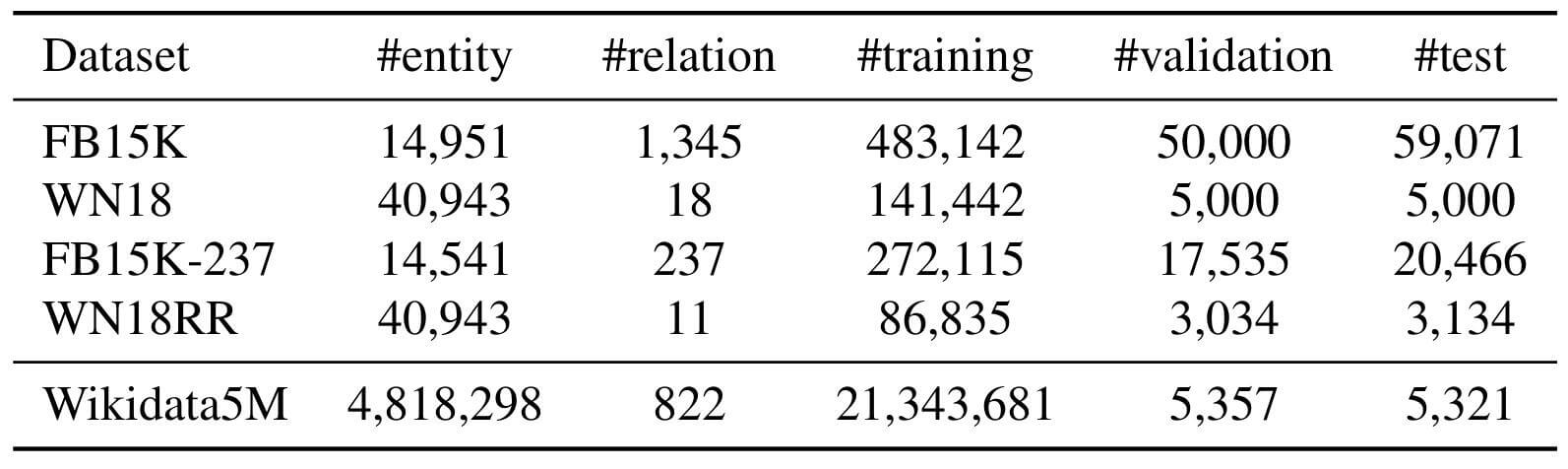Wikidata5M中实体类型统计: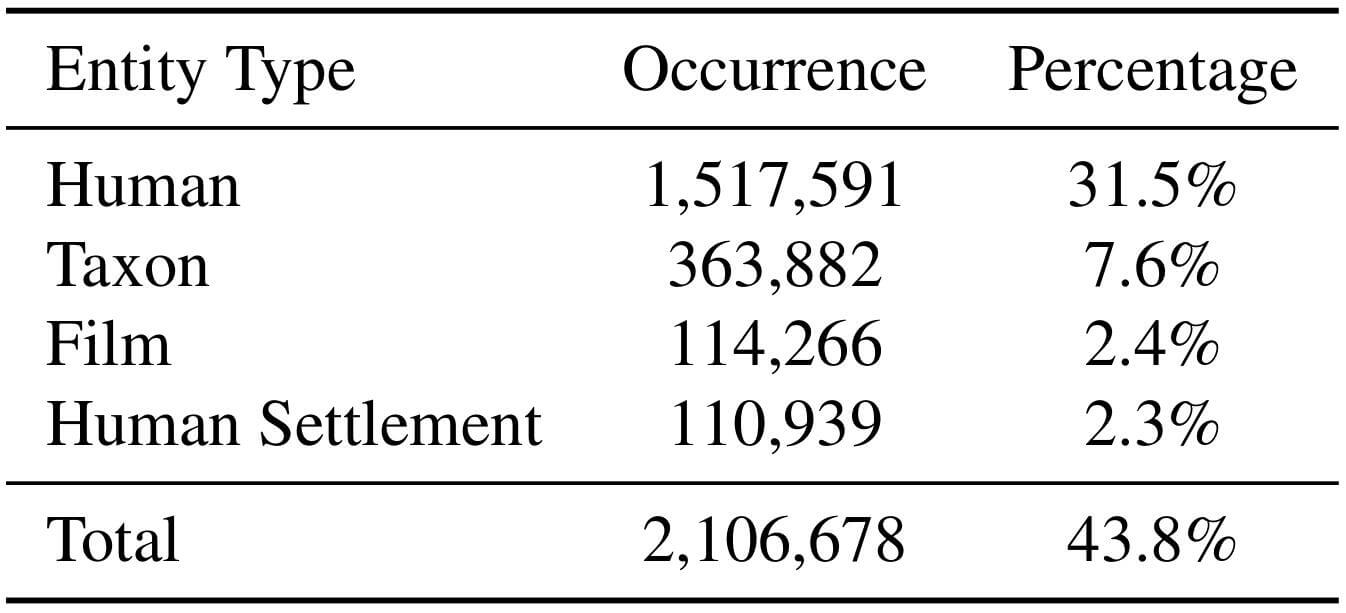##### DataSplit

• Transductive Setting: 在绝大多数KG数据集中使用, 对于训练集, 验证集, 测试集共享所有实体, 但并不知道完整三元组.

• Inductive Setting: 训练集, 验证集, 测试集中, 实体和三元组都不共享. 这更考验模型的推断能力, 也更困难. 但它更符合现实世界的应用情况. 具体数据集划分情况如下: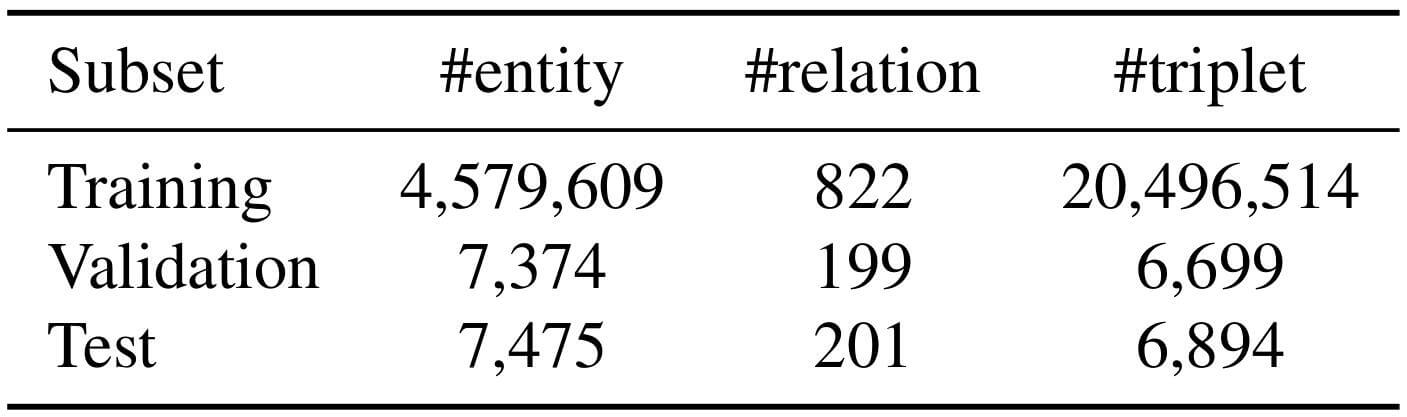##### Benchmark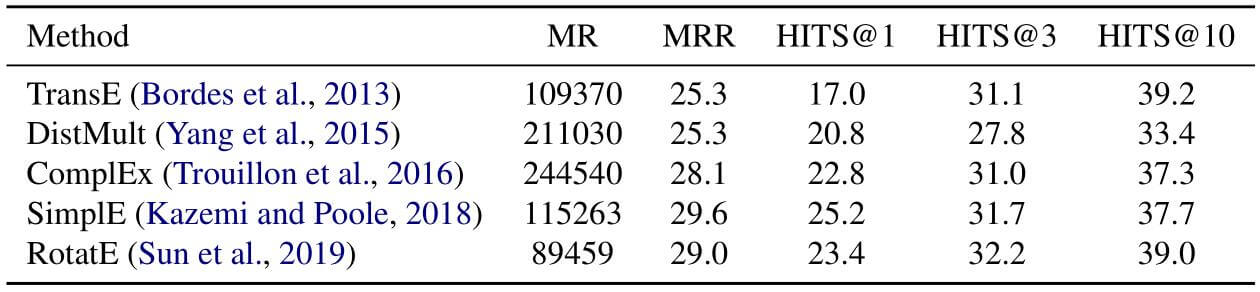#### MLM Objective

• BookCorpus.
• English WIkipedia.

### Pre - Training Settings

#### KE Settings

1. KEPLER - Wiki: 用Wikidata5M训练KEPLER, 总使用描述的前512个Token. 当使用实体和关系一起作为输入时(Using Entity and Relation Descriptions), 模型被称为KEPLER - Wiki - rel.
2. KEPLER - WordNet: 用WordNet训练KEPLER, 作者尝试将更多的语言知识融入进去, 或许会有益于NLP任务. WordNet中的关系数量相对来说非常少, 所以只采用实体描述.
3. KEPLER - W + W: 联合训练Wikidata5M和WordNet. 损失函数相应的发生变化:
$$\mathcal{L}=\mathcal{L}_{\mathrm{Wiki}}+ \mathcal{L}_{\mathrm{WordNet}}+\mathcal{L}_{\mathrm{MLM}}$$

#### RoBERTa

KEPLER是基于RoBERTa的, RoBERTa采用了更大的语料库进行训练. 为公平起见, 作者训练了RoBERTa*, 也是用RoBERTa的权重进行初始化, 但用相同的语料库, 并只对它的MLM目标继续优化.

#### Relation Classification

##### TACRED

TACRED是人为标注用于关系分类的数据集. 在TACRED上表现如下: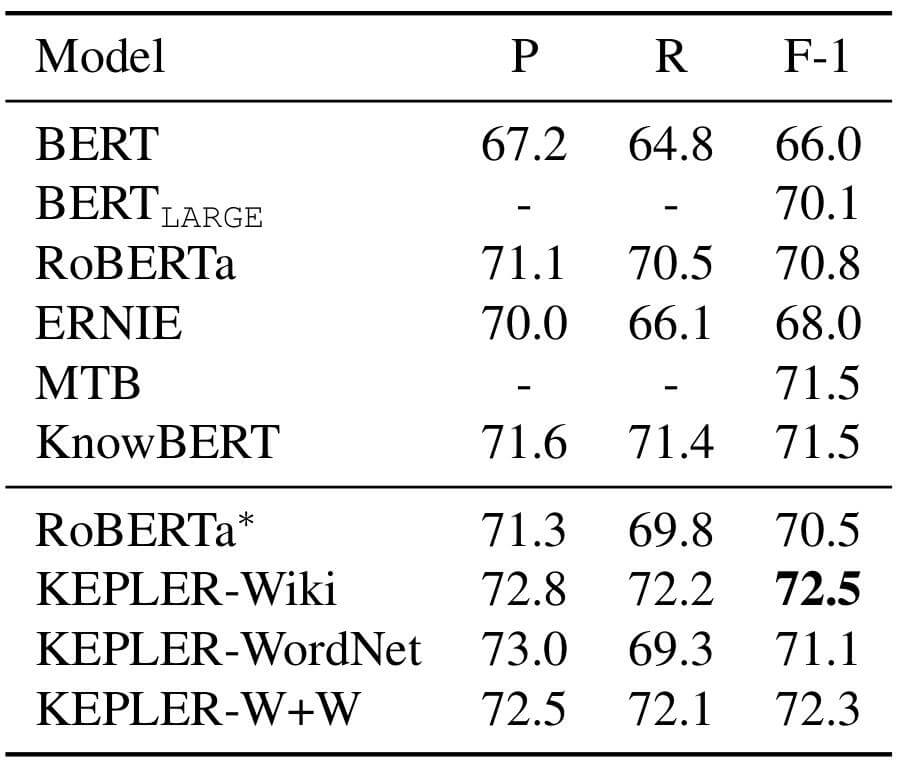KEPLER - Wiki表现很棒, 与RoBERTa*相比有很大进步, 但KEPLER - WordNet表现只比RoBERTa*好一点点. KEPLER - W + W和KEPLER - Wiki表现相当, 作者认为是WordNet限制了性能.

#### FewRel

FewRel是用于体现Few - Shot能力的关系分类数据集, 其2.0版本添加了更多的领域. 在FewRel上表现如下: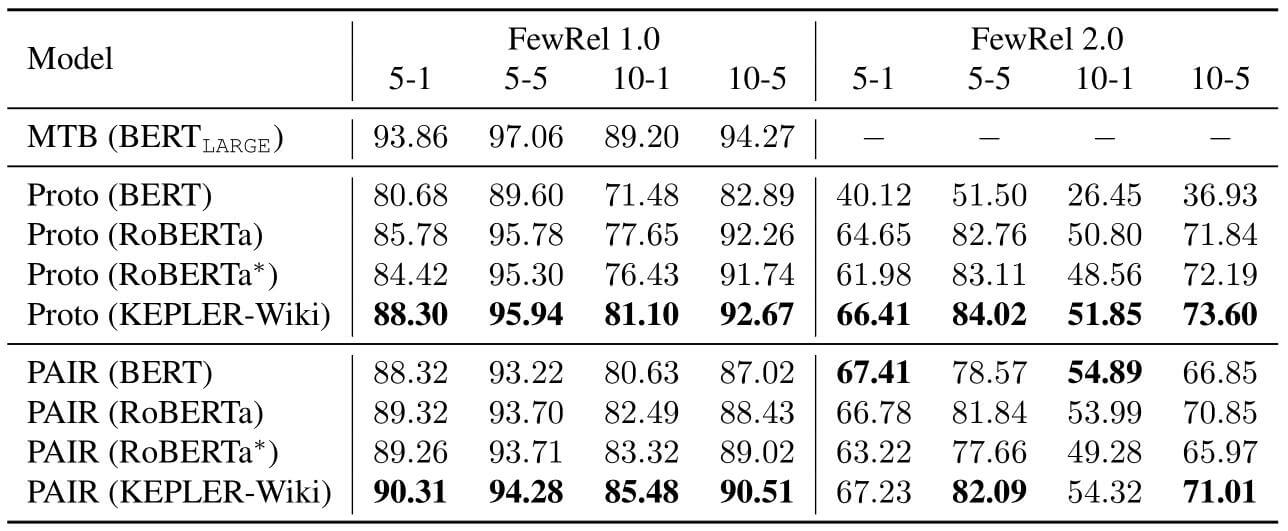#### Entity Typing

KEPLER在OpenEntity上的表现如下: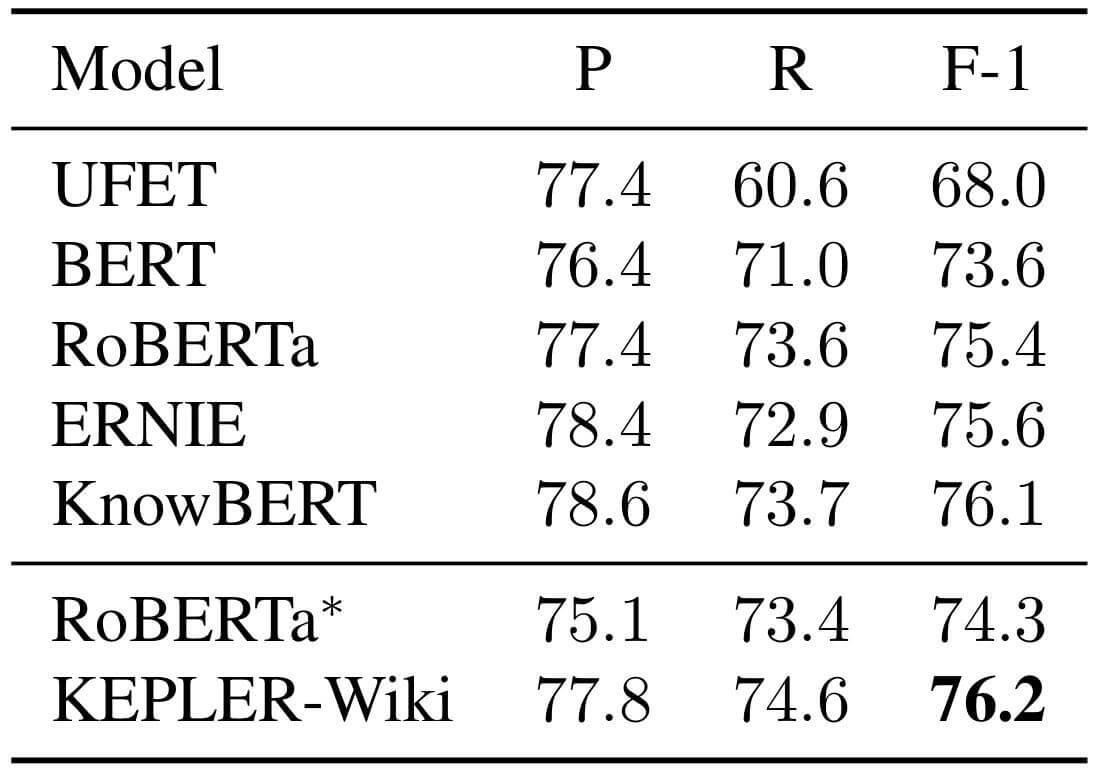#### GLUE

GLUE(General Language Understanding Evaluation)是用来评判语言理解能力的测试, 这种任务不需要知识, 但需要理解能力. 作者通过GLUE尝试证明KEPLER对NLU任务表现没有退化.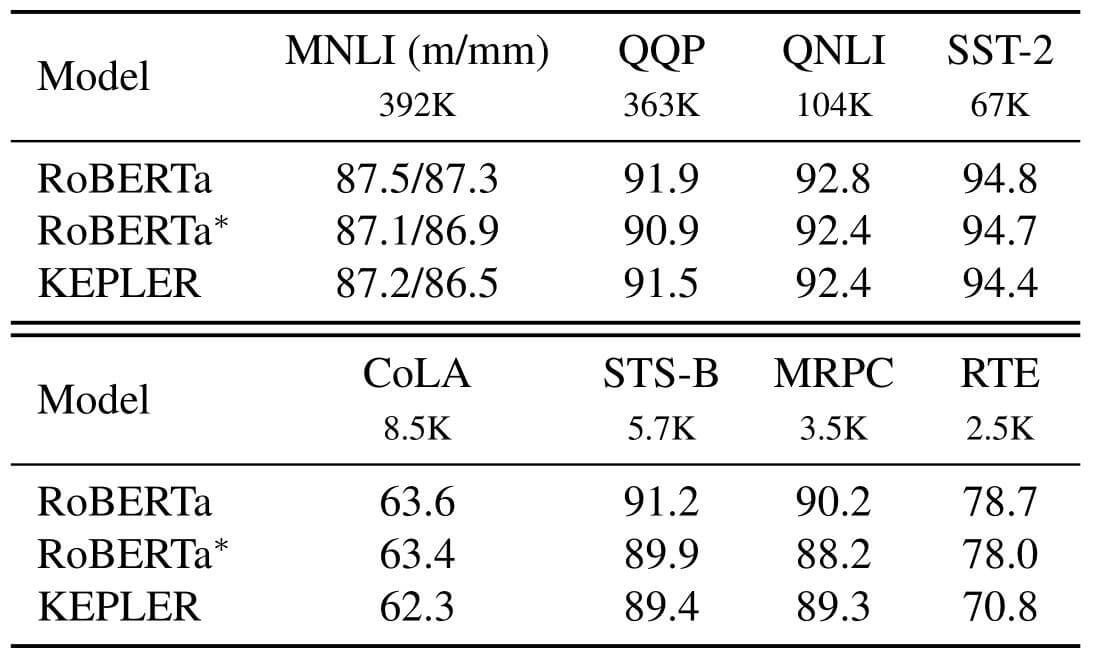#### Transductive Setting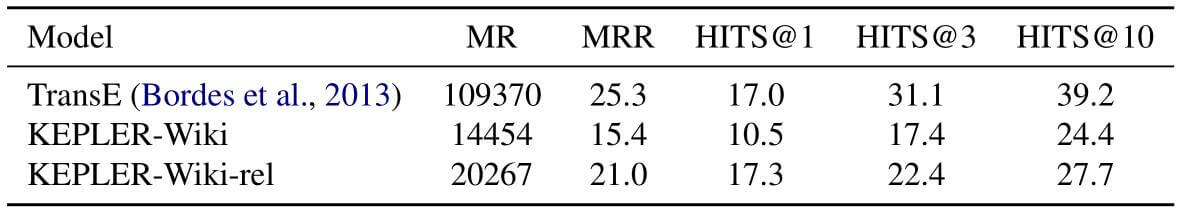#### Inductive Setting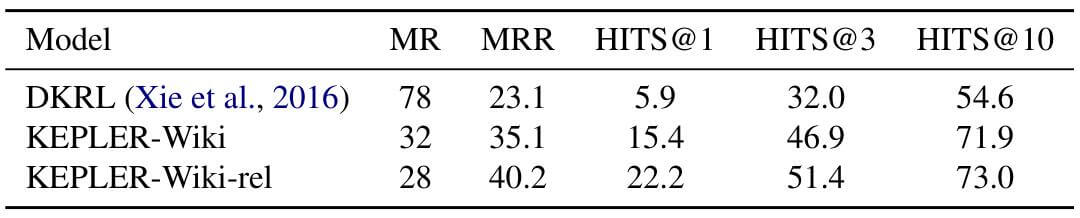KEPLER - Wiki - rel比KEPLER - Wiki要强大许多, 并且比DKRL提升巨大.

### Ablation Study

KEPLER是一个多任务学习的模型, 作者希望探究对KEPLER性能提升的因素.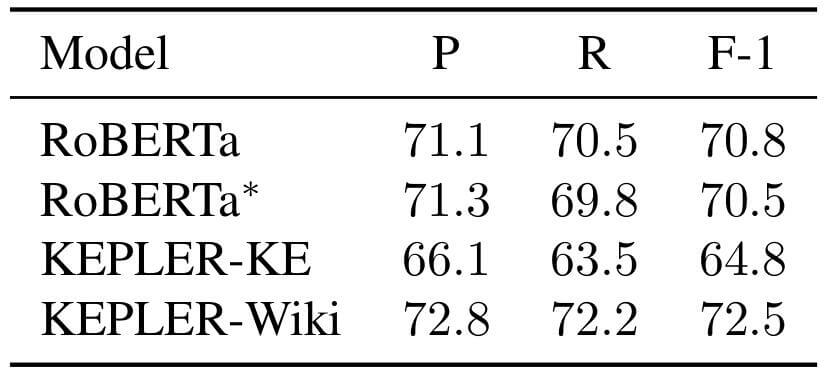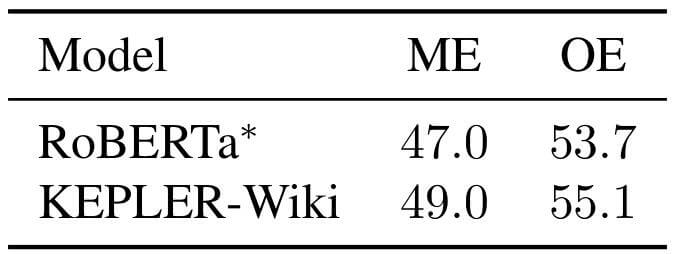KEPLER - Wiki学习到一些知识, 比用同等数据训练出来的RoBERTa*效果要更好一些.

## Summary

• KEPLER作为PLM, 将常识集成进了语言表示, 并且具有强大的语言理解能力, 并且增强了抽取知识的能力, 能够直接适应很多NLP任务.
• KEPLER作为KEM, 能够使用丰富的文本信息, 能在文本描述的引导下预测从没见过的实体.

上一篇Pytorch实现: Transformer

2020-11-23KGE预警论文两则

2020-11-20
目录